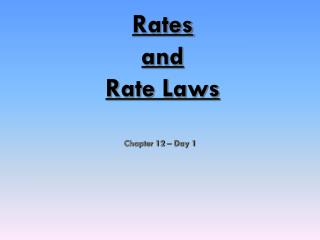DownloadDownload PresentationRates and Rate Laws

# Rates and Rate Laws

Download Presentation## Rates and Rate Laws

- - - - - - - - - - - - - - - - - - - - - - - - - - - E N D - - - - - - - - - - - - - - - - - - - - - - - - - - -
##### Presentation Transcript

1. RatesandRate Laws Chapter 12 – Day 1

2. Kinetics • The study of reaction rates. • Spontaneous reactions are reactions that will happen - but we can’t tell how fast. • Diamond will spontaneously turn to graphite – eventually. • Reaction mechanism- the steps by which a reaction takes place.

3. Reaction Rate The change in concentration of a reactant or product per unit of time

4. Reaction Rates: 2NO2(g)  2NO(g) + O2(g) 1. Can measure disappearance of reactants 2. Can measure appearance of products 3. Are proportional stoichiometrically

5. Reaction Rates: 2NO2(g)  2NO(g) + O2(g) 4. Are equal to the slope tangent to that point 5. Change as the reaction proceeds, if the rate is dependent upon concentration [NO2] t

6. Rate Laws Differential rate lawsexpress (reveal) the relationship between the concentration of reactants and the rate of the reaction. The differential rate law is usually just called “the rate law.” Integrated rate lawsexpress (reveal) the relationship between concentration of reactants and time

7. Writing a (differential) Rate Law Problem- Write the rate law, determine the value of the rate constant, k, and the overall order for the following reaction: 2 NO(g) + Cl2(g)  2 NOCl(g)

8. Writing a Rate Law Part 1– Determine the values for the exponents in the rate law: R = k[NO]x[Cl2]y In experiment 1 and 2, [Cl2] is constant while [NO] doubles. The rate quadruples, so the reaction is second order with respect to [NO]  R = k[NO]2[Cl2]y

9. Writing a Rate Law Part 1– Determine the values for the exponents in the rate law: R = k[NO]2[Cl2]y In experiment 2 and 4, [NO] is constant while [Cl2] doubles. The rate doubles, so the reaction is first order with respect to [Cl2]  R = k[NO]2[Cl2]

10. Writing a Rate Law Part 2– Determine the value for k, the rate constant, by using any set of experimental data: R = k[NO]2[Cl2]

11. Writing a Rate Law Part 3– Determine the overall order for the reaction. R = k[NO]2[Cl2] = 3 2 + 1  The reaction is 3rd order Overall order is the sum of the exponents, or orders, of the reactants

12. Determining Order withConcentration vs. Time data (the Integrated Rate Law) Zero Order: First Order: Second Order:

13. Solving an Integrated Rate Law Problem: Find the integrated rate law and the value for the rate constant, k A graphing calculator with linear regression analysis greatly simplifies this process!!

14. Time vs. [H2O2] Regression results: y = ax + b a = -2.64 x 10-4 b = 0.841 r2 = 0.8891 r = -0.9429

15. Time vs. ln[H2O2] Regression results: y = ax + b a = -8.35 x 10-4 b = -.005 r2 = 0.99978 r = -0.9999

16. Time vs. 1/[H2O2] Regression results: y = ax + b a = 0.00460 b = -0.847 r2 = 0.8723 r = 0.9340

17. And the winner is… Time vs. ln[H2O2] 1. As a result, the reaction is 1st order 2. The (differential) rate law is: 3. The integrated rate law is: 4. But…what is the rate constant, k ?

18. Finding the Rate Constant, k Method #1: Calculate the slope from the Time vs. ln[H2O2] table. Now remember:  k = -slope k = 8.32 x 10-4s-1

19. Finding the Rate Constant, k Method #2: Obtain k from the linear regresssion analysis. Regression results: y = ax + b a = -8.35 x 10-4 b = -.005 r2 = 0.99978 r = -0.9999 Now remember:  k = -slope k = 8.35 x 10-4s-1

20. Rate Laws Summary